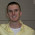### Advanced Analytics on Apache Spark

Developed in AMPLab at UC Berkeley, Apache Spark has become an increasingly popular platform to perform large scale analysis on Big Data. With run-times up to 100x faster than MapReduce, Spark is well suited for machine learning applications.

Spark is written in Scala but has APIs for Java and Python. As the NAG Library is accessible from both Java and Python, this allows Spark users access to over 1600 high quality mathematical routines. The NAG Library covers areas such as:
• Machine Learning including
• Linear regression (with constraints)
• Logistic regression (with constraints)
• Principal Component Analysis (A good article relating Machine Learning and PCA can be found here)
• Hierarchical cluster analysis
• K-means
• Statistics including
• Summary information (mean, variance, etc)
• Correlation
• Probabilities and deviates for normal, student-t, chi-squared, beta, and many more distributions
• Random number generation
• Quantiles
• Optimization including
• Linear, nonlinear, quadratic, and sum of squares for the objective function
• Constraints can be simple bounds, linear, or even nonlinear
• Matrix functions
• Inversion
• Nearest correlation
• Eigenvalues + eigenvectors

#### Calling the NAG Library on Spark

The fundamental datatype used in Spark is the Resilient Distributed Dataset (RDD). A RDD acts as a pointer to your distributed data on the filesystem. This object has intuitive methods (count, sample, filter, map/reduce, etc) and lazy evaluation that allow for fast and easy manipulation of distributed data.

Below is a simple Python example of using the NAG Library in Spark to calculate the cumulative Normal distribution function on a set of numbers (the message passing output from Spark has been omitted):

SparkContext available as sc
>>>  from nag4py.s import nag_cumul_normal
>>>  myNumbers = sc.parallelize( [-2.0, -1.0, 0.0, 1.0, 2.0] )
>>>  myNumbers.takeSample(False, 5, 0)
[ 0.0, -2.0, -1.0, 1.0, 2.0]

>>>  myNumbers.map( nag_cumul_normal ).takeSample(False, 5, 0)
[0.5, 0.02275, 0.15865, 0.84134, .97725]

It should be noted that the vast majority of the algorithms employed in the NAG library require all relevant data to be held in memory. This may seem to deviate from the Spark ecosystem, however when working with large datasets, two usage scenarios are commonly seen:
1. The full dataset is split into subsets, for example a dataset covering the whole world may be split by country, county and city and an independent analysis carried out on each subset. In such cases all the relevant data for a given analysis may be held on a single node and therefore can be processed directly by NAG library routines.
2. A single analysis is required that utilizes the full dataset. In this case it is sometimes possible to reformulate the problem. For example many statistical techniques can be reformulated as a maximum likelihood optimization problem. The objective function of such an optimization (the likelihood) can then be evaluated using the standard Spark map/reduce functions and the results fed back to one of the robust optimization routines available in the NAG library.

For more information on using the NAG Library in Spark or any of the other topics touched upon in this article please contact NAG at support@nag.com.

1.Hi,
I'm dealing with Image Processing algorithms.
One of our algorithm requires solving large scale Eigen Value system.

The problem is the matrix size is the number of pixels of the image by the patch size, which might be huge.

We're looking for algorithms to solve such problems yet dealing with practical memory sources (Above 2 GB yet below 4GB).

I was wondering if you have algorithm optimized for those scenarios.
Those matrices are usually sparse and we're looking for algorithm to solve them without requiring the inversion of the whole matrix.

1.Hi Royi,

Depending upon the specific's of your problem (whether the matrix is symmetric, banded, etc), we might have an algorithm suitable. I would recommend looking at the f12 Chapter:

http://www.nag.com/numeric/CL/nagdoc_cl24/html/F12/f12intro.html

You can email support@nag.com to receive trial licenses of the NAG Library to test algorithms.

2.This comment has been removed by the author.

3.Hi,
We are looking for Spark solution for performing non linear optimization along with both linear and non linear constraints.
Currently we are providing solution using SAS and Lindo. However, since we are looking for large scale optimization problems we would want to leverage distributed computing to provide the solution.
Can you help me with my requirement on how to use Apache Spark.
TIA!

4.Hi Jyoti. If you could email us at sales@nag.co.uk with your enquiry we can discuss your particular requirements. Kind regards.